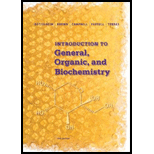# 2-25 Name and give the symbol for each element in Problem 2-24.### Introduction to General, Organic a...

11th Edition
Frederick A. Bettelheim + 4 others
Publisher: Cengage Learning
ISBN: 9781285869759### Introduction to General, Organic a...

11th Edition
Frederick A. Bettelheim + 4 others
Publisher: Cengage Learning
ISBN: 9781285869759

#### Solutions

Chapter
Section
Chapter 2, Problem 2.25P
Textbook Problem
1 views

## 2-25 Name and give the symbol for each element in Problem 2-24.

Expert Solution
Interpretation Introduction

(a)

Interpretation:

The name and symbol of an atom having 22 protons, 22 electrons and 26 neutrons should be identified.

Concept Introduction:

Each element has a specific atomic number which is the identity of that element.

### Explanation of Solution

Atomic number is the number of protons present in the nucleus that determines the identity of the element. Since, the given element has...

Expert Solution
Interpretation Introduction

(b)

Interpretation:

The name and symbol of an atom having 76 protons, 76 electrons and 114 neutrons should be identified.

Concept Introduction:

Each element has a specific atomic number which is the identity of that element.

Expert Solution
Interpretation Introduction

(c)

Interpretation:

The name and symbol of an atom having 34 protons, 34 electrons and 45 neutrons should be identified.

Concept Introduction:

Each element has a specific atomic number which is the identity of that element.

Expert Solution
Interpretation Introduction

(d)

Interpretation:

The name and symbol of an atom having 94 protons, 94 electrons and 150 neutrons should be identified.

Concept Introduction:

Each element has a specific atomic number which is the identity of that element.

### Want to see the full answer?

Check out a sample textbook solution.See solution

### Want to see this answer and more?

Bartleby provides explanations to thousands of textbook problems written by our experts, many with advanced degrees!

See solution